Students can track their progress and improvement through regular use of ICSE S Chand Maths Class 9 Solutions Chapter 20 Coordinates and Graphs of Simultaneous Linear Equations Ex 20(B)

## S Chand Class 9 ICSE Maths Solutions Chapter 20 Coordinates and Graphs of Simultaneous Linear Equations Ex 20(B)

Question 1.
Fill in the blanks :
(i) The graph of x = 1 is a line parallel to the ………. axis.
(ii) The graph of y = 1 is a line parallel to the ………. axis.
(iii) The equation ax + by + c = 0 (where a and b are not both zero) is called ……….. equation.
(iv) The graph of 2x = 1 is a line parallel to the ………. axis.
Solution:
(i) The graph of x = 1 is a line parallel to the y-axis.
(ii) The graph of y = 1 is a line parallel to the x- axis.
(iii) The equation ax + by + c = 0 (where a and b are not both zero) is called linear equation.
(iv) The graph of 2x = 1 is a line parallel to the y- axis.Question 2.
Graph the following equations :
(i) x = 3
Solution:
As x = 3 is a line parallel is y-axis at a distance of 3 units
Now the equation x = 3 can be written as x + 0 y = 3
Giving any values of y as 1,2, 3, etc. we get x = 3
Now plot the points (3, 1),(3, 2),(3, 3), ………… on the graph and join them to get the required line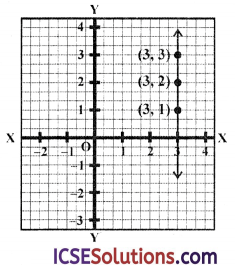(ii) y = -4
Solution:
As y = -4 is a line parallel to x-axis at a distance of -4 units. We can write this equation as 0x + y = 4
Now giving any values to x say 1,2,-1 we get y = -4
Now plot the points is (1,-4),(2,-4)(-1, -4). On the graph and join than to get the required line.(iii) 2x = -7
Solution:
As 2 x = -7 ⇒ x =$$\frac{-7}{2}$$ is a line parallel y-axis at a distance of $$\frac{-7}{2}$$. We can write it as 2x +0y = -7
Now giving some values to 1,2,3 we get 2x = -7
Now plot the points , $$\left(\frac{-7}{2}, 1\right)$$, $$\left(\frac{-7}{2}, 3\right)$$ on the graph and join them to get the required line.(iv) y = 2 x
Solution:
y = 2 x
By giving some value to x we get the corresponding values of y as shown in the following table

 X 1 2 -1 y 2 4 -2

Now plot the points (1, 2),(2, 4) and (-1, -2) on the graph and join them to get the required line.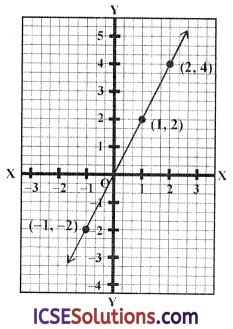(v) y = -3x
Solution:
y = -3x
By giving some values to x, we get the corresponding values of y as given below :

 X 1 0 -1 y -3 0 3

Now plot the points (1,-3),(0,0) and (-1, 3 ) on the graph and join them to get the required line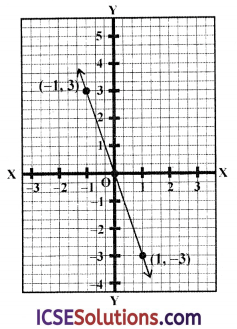(vi) y = x + 1
Solution:
y = x + 1
By giving some values to x we get the corresponding values of y as shown below :

 X 1 0 -1 y 2 1 0Now plot the points (1,2),(0,1) and (-1, 0 ) on the graph and join them to get the required line

(vii) 2x + y = 14
Solution:
2x + y = 14
⇒ y = 14 – 2x
Giving some different values to x, we get corresponding values of y as given below :

 X 5 6 7 y 4 2 0

Now plot the points (5, 4),(6, 2) and (7, 0) on the graph and join them to get the required line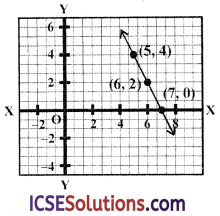(viii) 4x + 3y = 6
Solution:
4x + 3y = 6
⇒ 4x = 6 – 3y
⇒ x = $$\frac{6-3 y}{4}$$
Giving some different values to y we get corresponding values of x as given below :

 X 0 -3 3 y 2 6 -2

Now plot the points (0,2),(-3,6) and (3, -2 ) on the graph and join them to get the required line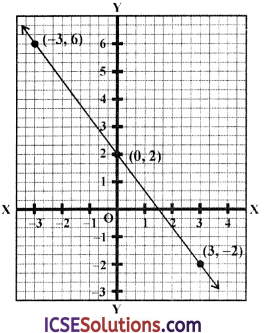(ix) x = 3y + 1
Solution:
x = 3y + 1
Giving some different values to y, we get corresponding values of x as given below :

 X 1 4 -2 y 0 1 -1

Now plot the points (1, 0),(4, 1) and (-2, -1) on the graph and join them to get the required line.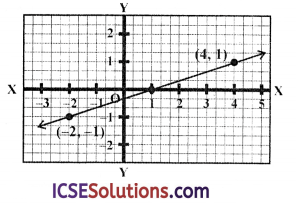Question 3.
Draw the graph of equation y = 3x – 4. Find graphically
(i) the values of y, when x = -1.
(ii) the value of x when y = 5.
Solution:
y = 3x – 4
Giving some suitable value to x, we get the corresponding values of y as shown below:

 X 0 1 2 y -4 -1 2

Now plot the points (0, -4),(1, -1) and (2, 2) on the graph and join than to get the required line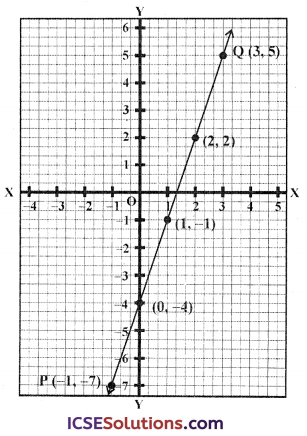(i) From x = -1, draw a perpendicular which meet the line at P. From P draw a line || to x- axis meeting y-axis at -7
∴ If x = -1, then y = -7

(ii) Similarly from x = 5, draw a perpendicular on y-axis which meets the line at Q From Q, draw perpendicular on x-axis meeting at 3
∴ x = 3, y = 5Question 4.
Find the coordinates of the point where the following lines cut the y-axis.
(i) y = 5x + 1,
(ii) y = 3x – 7
(iii) y = x + 5,
(iv) 3y = 2x + 9.
Solution:
We know that a line cuts the y-axis when x = 0
(i) ∴ y = 5 x + 1 cut the y-axis, when x = 0, When x = 0, then y = 5 × 0 + 1 = 0 + 1 = 1
∴ y = 1
∴ It will cut y-axis at (0, 1)

(ii) y = 3x – 7 will cut the y-axis when x = 0
Now, if x = 0, then y = 3 × 0 – 7 = 0 – 7 = -7
∴ It will cut y-axis at (0, -7)

(iii) y = x + 5 will cut y-axis when x = 0,
Now if x = 0, then y = 0 + 5 = 5
∴ It will cut y-axis at (0, 5)

(iv) 3y = 2x + 9 will cut y-axis when x = 0
Now if x = 0, then 3y = 2 × 0 + 9
⇒ 3y = 0 + 9 = 9 ⇒ y = $$\frac { 9 }{ 3 }$$ = 3
∴ It will cut y-axis at (0, 3)